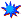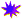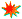Mountain Shadows Phonemic Awarenss Scale (MS-PAS)
Score Sheet
MS-PAS Page 1
MS-PAS Page 2
MS-PAS Page 3
MS-PAS Page 4
MS-PAS Page 5
MS-PAS Page 6

Watkins, M. W., & Edwards, V. A. (2004). Assessing early literacy skills with the Mountain Shadows Phonemic Awareness Scale (MS-PAS). Journal of Psychoeducational Assessment, 22, 3-14.

Nelson, J. M. (2008). Psychometric evaluation of the Mountain Shadows Phonemic Awareness Scale with a kindergarten sample. Journal of Psychoeducational Assessment, 26, 287-298.Psychoeducational report, recall measure, and satisfaction measure (in pdf format) from: Miller, J. A., & Watkins, M. W. The use of graphs to communicate psychoeducational test results to parents.Watkins, M. W., & Browning, L. J. (2015). The Baylor revision of the Motivation to Read Survey (B-MRS). Research and Practice in the Schools,  3, 37-50.

### All programs 64 bit as of April, 2020

 Link Title Description Mac Windows ASCA Assistant A standalone scoring program for the Adjustment Scales for Children and Adolescents (ASCA). It also performs syndromic profile analysis, discriminant analysis, and situtype analysis. Only for those who own ASCA manual and protocols. Mac Windows MacParallel Analysis A standalone program that contains tables of eigenvalues produced by Monte Carlo simulations for determination of Parallel Analysis criteria. Mac Windows Monte Carlo PCA for Parallel Analysis A standalone program that computes Parallel Analysis criteria (eigenvalues) by performing a Monte Carlo simulation based on the number of subjects, variables, and repetitions. Mac Windows r To z Conversion A standalone program that calculates r-to-z and z-to-r. Mac Windows Ability-Achievement Discrepancy Analysis A standalone program that performs a simple regression-based prediction of achievement for determination of a severe ability-achievement discrepancy. Mac Windows Rc Coefficient of Congruence A standalone program that calculates the coefficient of congruence for comparing factor solutions from different studies or different samples. Mac Windows Diagnostic Utilitity Statistics A standalone program that calculates diagnostic efficiency statistics: sensitivity, specificity, false positive rate, false negative rate, positive and negative predictive power, odds ratio, Kappa, AUC, etc. Mac Windows ROC Analysis A standalone program that graphs the Receiver Operating Characteristic (ROC) curve and calculates the Area Under the ROC Curve (AUC) using a nonparametric method. Mac Windows Chi-Square Analysis A standalone program that calculates the chi-square statistic for 2x2 to 10x10 contingency tables. Also calculates the contingency, phi, and kappa statistics. Mac Windows Probability Calc A standalone program that calculates the probability of z, t, F, and chi-square values. Mac Windows MacBonferroni A standalone program that calculates Holm's sequential Bonferroni method to maintain experimentwise error rates at a desired alpha level. Mac Windows RandomNum A standalone program that generates random numbers from uniform and normal distributions. Mac Windows RangeRestrict A standalone program that computes the correlation when the variability of scores in a sample is smaller than that found in the population. Mac Windows CorrectAttenuation A standalone program that estimates the correlation that would be obtained if two perfectly reliable measures had been used. Mac Windows Simple t A standalone program that calculates independent and dependent t-tests from group data (mean, standard deviation, correlation). Mac Windows MacKappa A standalone program that calculates general and partial kappa coefficients for nominal data. Mac Windows CAcalculator A standalone program that calculates chronological age when given date of birth and date of test. Mac Windows rProphecy A standalone program that calculates the Spearman-Brown 'Prophecy' formula that estimates the reliability of a test if its number of items is altered. Mac Windows Effect Size Calculator A standalone program that calculates effect sizes (Cohen's d, r, Glass's Δ, Common Language Effect Size) given appropriate means and standard deviations. Mac Windows MacOrtho A standalone program that transforms an hierarchical higher-order factor analysis solution into an orthogonal solution as described by Schmid and Leiman (1957). Mac Windows MacMahalanobis A standalone program that calculates Mahalanobis distance for multiple cases, given entry of an inverted covariance matrix. Mac Windows Pairwise Tests A standalone program that calculates three Pairwise Tests: simple Bonferroni adjustment, Holm's sequential Bonferroni method, and Benjamin and Hochberg's False Discovery Rate. Mac Windows alphaN A standalone program that estimates the sample size needed for a specified coefficient alpha, given the Type I error rate and effect size. Mac Windows rCI A standalone program that computes the confidence interval of a correlation coefficient. Mac Windows rSim A standalone program that interactively simulates how the 95% confidence interval and statistical significance (p ≠ 0) of the correlation coefficient vary as a function of sample size. Mac Windows Invariance A standalone program that calculates three indices of factorial invariance: the coefficient of congruence, a chi-square goodness of fit test, and Cattell's Salient Variable Similarity index. Mac Windows MacPotthoff A standalone program that performs Potthoff regression bias analysis. Mac Windows MacRCI A standalone program that calculates the Reliable Change Index (RCI) of Jacobson and Truax (1991). Mac Windows Standard Score Converter A standalone program that converts raw scores to standard scores and converts between standard scores, including z, T, deviation IQ, NCE, percentile, etc. Mac Windows BayesianCalc A standalone program that calculates positive predictive power, given prevalence and test results. Mac Windows SEscree A standalone program that quantifies the visual scree test to determine the number of factors to retain in exploratory factor analysis. Mac Windows SimpleStatTests A standalone program that calculates tests of dependent and independent means, variances, correlations, proportions, alpha coefficients, and AUCs from group data. Mac Windows Reliability of Difference Scores A standalone program that calculates the reliability of difference scores, given the reliability of two tests and the correlation between those two tests. Mac Windows BMI Calculator A standalone program that calculates the Body Mass Index for adults given height and weight. Mac Windows CIeigenvalue A standalone program that computes the confidence intervals of obtained eigenvalues as per Larsen and Warne (2010). Mac Windows EDPcalc A standalone program for evidence-based practice that computes posterior probability when given prior probability and likelihood ratio. Mac Windows ChiSquareDiff A standalone Macintosh program to compute the statistical significance of chi-square difference tests as typically used in comparisons of nested SEM models in EQS, LISREL, and Mplus SEM software as per Bryant and Satorra (2012). Mac Windows Omega A standalone program to compute omega and omega hierarchical, measures of measurment precision or reliability, using standardized loadings from a confirmatory factor analysis. Also includes ECV, H, and PUC values in addition to omega. Mac Windows alpha A standalone program to compute the alpha coefficient of internal consistency. Mac Windows CoinFlip A standalone program that uses a random number generator to simulate coin flips. Mac Windows MaxR A standalone program that computes the maximum validity coefficient given the reliability of two tests. Mac Structure Coefficients A standalone program that computes the structure coefficient matrix from the pattern matrix and the matrix of factor intercorrelations. Mac Windows Higher-Order A standalone program that computes the loading of a second-order factor onto the observed variables given the pattern coefficients from first-order factoring. Mac Windows Partial Correlation A standalone program that computes the partial correlation given reliability and correlation. Mac Windows Multiple Correlation A standalone program that computes the multiple correlation and squared multiple correlation of each variable with all the other variables in a correlation matrix. Mac RegPart A standalone program that computes Pratt's measure of the relative importance of predictor variables in linear regression. Mac Cor<>Cov A standalone program that converts correlation matrices to covariance matrices and vice versa. Mac AIC A standalone program that computes AIC difference, Akaike weights, and Akaike evidence ratios. Mac Windows BIC A standalone program that identifies the most plausible model using BIC posterior and BIC factor values. Mac Windows ICLbic A standalone program that computes the Integrated Classification Likelihood with Bayesian-type Approximation (ICLbic; Biernacki, Celeux, & Govaert, 2000). Mac Windows Visual Bayes A standalone program that visually displays a Bayesian approach to positive predictive power, given prevalence and test results. Mac Windows Polya's Urn A standalone program that displays a simulation of Polya's urn: a sampling distribution demonstration that illustrates how small imbalances can be magnified over time. Mac Windows Residuals A standalone program that describes a residual matrix in terms of RMSR and numbers of residuals >.05 and >.10. Mac Windows Multicollinearity A standalone program to compute Haitovsky's (1969) test of singularity for a correlation matrix. If statistically significant, the correlation matrix is not suitable for further statistical manipulation.(Click on the Mac for Macintosh and Windows for Windows operating system versions)